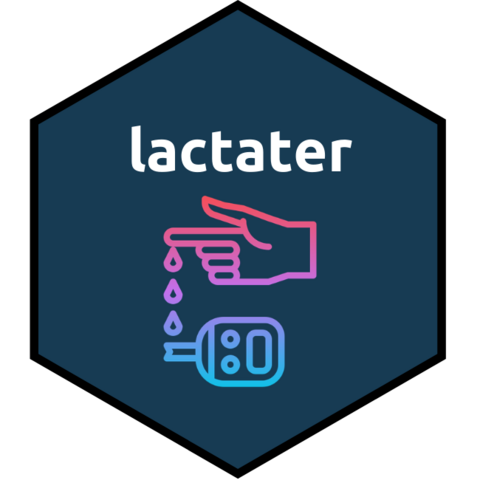# lactaterThe goal of `lactater` is to provide tools for making it easier to analyze lactate thresholds.

## Installation

You can install the released version of `lactater` from CRAN with:

``install.packages("lactater")``

You can install the development version of `lactater` from Github with:

``````# install.packages("remotes")
remotes::install_github("fmmattioni/lactater")``````

## Demo data

``````library(lactater)

demo_data
#>   step length intensity lactate heart_rate
#> 1    0      0         0    0.93         96
#> 2    1      3        50    0.98        114
#> 3    2      3        75    1.23        134
#> 4    3      3       100    1.88        154
#> 5    4      3       125    2.80        170
#> 6    5      3       150    4.21        182
#> 7    6      3       175    6.66        193
#> 8    7      2       191    8.64        198``````

## Usage

With `lactater` you can easily estimate lactate thresholds using one or multiple methods:

``````results_overall <- lactate_threshold(
.data = demo_data,
intensity_column = "intensity",
lactate_column = "lactate",
heart_rate_column = "heart_rate",
method = c("Log-log", "OBLA", "Bsln+", "Dmax", "LTP", "LTratio"),
fit = "3rd degree polynomial",
include_baseline = TRUE,
sport = "cycling",
plot = TRUE
)``````
``````#> # A tibble: 17 × 7
#>    method_category method           fitting   intensity lactate heart_rate plot
#>    <fct>           <fct>            <chr>         <dbl>   <dbl>      <dbl> <lis>
#>  1 Log-log         Log-log          3rd degr…      68.3    1.2         131 <gg>
#>  2 OBLA            OBLA 2.0         3rd degr…     105.     2           153 <gg>
#>  3 OBLA            OBLA 2.5         3rd degr…     118.     2.5         160 <gg>
#>  4 OBLA            OBLA 3.0         3rd degr…     129      3           167 <gg>
#>  5 OBLA            OBLA 3.5         3rd degr…     137      3.5         171 <gg>
#>  6 OBLA            OBLA 4.0         3rd degr…     145      4           176 <gg>
#>  7 Bsln+           Bsln + 0.5       3rd degr…      82.5    1.43        139 <gg>
#>  8 Bsln+           Bsln + 1.0       3rd degr…     104.     1.93        152 <gg>
#>  9 Bsln+           Bsln + 1.5       3rd degr…     117.     2.43        159 <gg>
#> 10 Dmax            Dmax             3rd degr…     132.     3.1         168 <gg>
#> 11 Dmax            ModDmax          3rd degr…     140.     3.6         173 <gg>
#> 12 Dmax            Exp-Dmax         Exponent…     135.     3.3         170 <gg>
#> 13 Dmax            Log-Poly-ModDmax 3rd degr…     137.     3.4         172 <gg>
#> 14 Dmax            Log-Exp-ModDmax  Exponent…     141.     3.6         174 <gg>
#> 15 LTP             LTP1             3rd degr…      88.9    1.5         143 <gg>
#> 16 LTP             LTP2             3rd degr…     148.     4.1         178 <gg>
#> 17 LTratio         LTratio          B-Spline…      71.2    1.2         132 <gg>``````## You can also choose one method:

### Log-log

``````results_loglog <- lactate_threshold(
.data = demo_data,
intensity_column = "intensity",
lactate_column = "lactate",
heart_rate_column = "heart_rate",
method = "Log-log",
fit = "3rd degree polynomial",
include_baseline = TRUE,
sport = "cycling",
plot = TRUE
)``````
``````#> # A tibble: 1 × 7
#>   method_category method  fitting             intensity lactate heart_rate plot
#>   <fct>           <fct>   <chr>                   <dbl>   <dbl>      <dbl> <lis>
#> 1 Log-log         Log-log 3rd degree polynom…      68.3     1.2        131 <gg>``````### OBLA

``````results_obla <- lactate_threshold(
.data = demo_data,
intensity_column = "intensity",
lactate_column = "lactate",
heart_rate_column = "heart_rate",
method = "OBLA",
fit = "3rd degree polynomial",
include_baseline = TRUE,
sport = "cycling",
plot = TRUE
)``````
``````#> # A tibble: 5 × 7
#>   method_category method   fitting            intensity lactate heart_rate plot
#>   <fct>           <fct>    <chr>                  <dbl>   <dbl>      <dbl> <lis>
#> 1 OBLA            OBLA 2.0 3rd degree polyno…      105.     2          153 <gg>
#> 2 OBLA            OBLA 2.5 3rd degree polyno…      118.     2.5        160 <gg>
#> 3 OBLA            OBLA 3.0 3rd degree polyno…      129      3          167 <gg>
#> 4 OBLA            OBLA 3.5 3rd degree polyno…      137      3.5        171 <gg>
#> 5 OBLA            OBLA 4.0 3rd degree polyno…      145      4          176 <gg>``````### Bsln+

``````results_bsln_plus <- lactate_threshold(
.data = demo_data,
intensity_column = "intensity",
lactate_column = "lactate",
heart_rate_column = "heart_rate",
method = "Bsln+",
fit = "3rd degree polynomial",
include_baseline = TRUE,
sport = "cycling",
plot = TRUE
)``````
``````#> # A tibble: 3 × 7
#>   method_category method     fitting          intensity lactate heart_rate plot
#>   <fct>           <fct>      <chr>                <dbl>   <dbl>      <dbl> <lis>
#> 1 Bsln+           Bsln + 0.5 3rd degree poly…      82.5    1.43        139 <gg>
#> 2 Bsln+           Bsln + 1.0 3rd degree poly…     104.     1.93        152 <gg>
#> 3 Bsln+           Bsln + 1.5 3rd degree poly…     117.     2.43        159 <gg>``````### Dmax

``````results_dmax <- lactate_threshold(
.data = demo_data,
intensity_column = "intensity",
lactate_column = "lactate",
heart_rate_column = "heart_rate",
method = "Dmax",
fit = "3rd degree polynomial",
include_baseline = TRUE,
sport = "cycling",
plot = TRUE
)``````
``````#> # A tibble: 5 × 7
#>   method_category method           fitting    intensity lactate heart_rate plot
#>   <fct>           <fct>            <chr>          <dbl>   <dbl>      <dbl> <lis>
#> 1 Dmax            Dmax             3rd degre…      132.     3.1        168 <gg>
#> 2 Dmax            ModDmax          3rd degre…      140.     3.6        173 <gg>
#> 3 Dmax            Exp-Dmax         Exponenti…      135.     3.3        170 <gg>
#> 4 Dmax            Log-Poly-ModDmax 3rd degre…      137.     3.4        172 <gg>
#> 5 Dmax            Log-Exp-ModDmax  Exponenti…      141.     3.6        174 <gg>``````### LTP

``````results_ltp <- lactate_threshold(
.data = demo_data,
intensity_column = "intensity",
lactate_column = "lactate",
heart_rate_column = "heart_rate",
method = "LTP",
fit = "3rd degree polynomial",
include_baseline = TRUE,
sport = "cycling",
plot = TRUE
)``````
``````#> # A tibble: 2 × 7
#>   method_category method fitting              intensity lactate heart_rate plot
#>   <fct>           <fct>  <chr>                    <dbl>   <dbl>      <dbl> <lis>
#> 1 LTP             LTP1   3rd degree polynomi…      88.9     1.5        143 <gg>
#> 2 LTP             LTP2   3rd degree polynomi…     148.      4.1        178 <gg>``````### LTratio

``````results_ltratio <- lactate_threshold(
.data = demo_data,
intensity_column = "intensity",
lactate_column = "lactate",
heart_rate_column = "heart_rate",
method = "LTratio",
fit = "3rd degree polynomial",
include_baseline = TRUE,
sport = "cycling",
plot = TRUE
)``````
``````#> # A tibble: 1 × 7
#>   method_category method  fitting            intensity lactate heart_rate plot
#>   <fct>           <fct>   <chr>                  <dbl>   <dbl>      <dbl> <list>
#> 1 LTratio         LTratio B-Spline (default)      71.2     1.2        132 <gg>``````